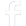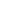#### WhatsApp Quick Chat#### Get Instant Call Back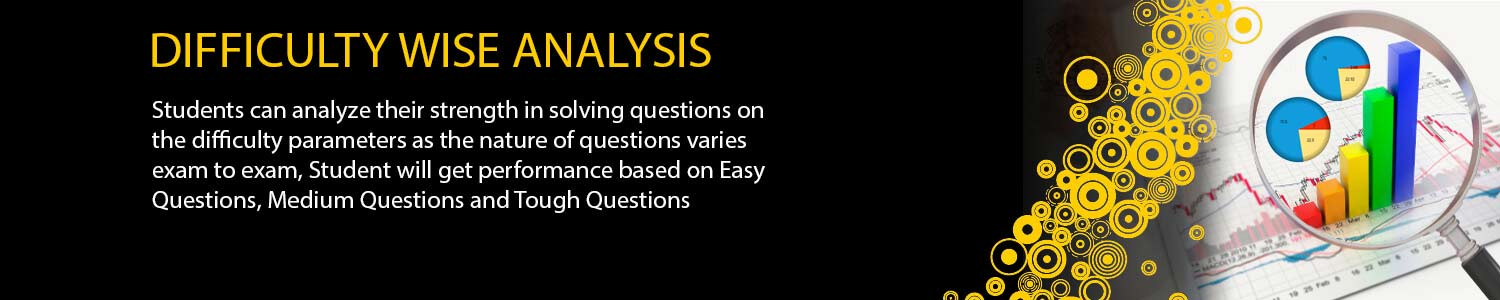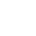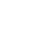## Offers## News & Events# JAM Maths Online Test Series

If you want to crack any competitive examination, then not only the understanding of the concept, but also practicing of the numerous numbers of questions relative to those concepts is necessary. You can divide it into two parts among them one part is preparing well for Exam and implementation of that knowledge and shortcuts on the exam day is another part. A large number of students become unsuccessful due to the lack of exam temperament and adoption of wrong approach on the exam day.

To overcome these obstacles students should have joined the test series, which gives them proper exam temperament and guide them according to their performance in these mock tests so that students become habitual of these kind of temperament and do not caught by nervousness at the time of exam.

Our test is designed in such a way so that you can get a large number of standard questions on every minute concept and provides proper exam temperament. This student becomes habitual to solve that number of questions in the given time and since our format of exam is same as per the exam conducting body so it helps them to perform well on the exam day.

##### Main features

Quality of the question as per the norms of Exam conducting body.

Question papers are designed as per latest exam's pattern.

You can get question on each and every concept which helps them in understanding the whole concept thoroughly.

Freedom to avail the test Topic/Concept wise and full length

Your whole performance is analyzed and displayed to you

Get Answer and solution post test

Question Papers are true copy of actual exam pattern.

Can give the test without leaving the comfort of your home#### JAM All India Ranker

(Online Test Series)#### JAM Real Time

(Online Test Series)#### JAM Anytime

(Online Test JAM)

## IIT-JAM MATH TEST SERIES 2020

# DATE TEST TYPE MODULES Syllabus
1 3-Jan-20 MWT-01 Group Theory Groups, subgroups, Abelian groups, non-abelian groups, cyclic groups, permutation groups; Normal subgroups, Lagrange's Theorem for finite groups, group homomorphisms and basic concepts of quotient groups (only group theory).
2 6-Jan-20 MWT-02 Integral Calculus Integration as the inverse process of differentiation, definite integrals and their properties, Fundamental theorem of integral calculus. Double and triple integrals, change of order of integration. Calculating surface areas and volumes using double integrals and applications. Calculating volumes using triple integrals and applications.
3 9-Jan-20 MWT-03 Sequences and Series of Real Numbers and LCD Sequences and series of real numbers, Convergent and divergent sequences, bounded and monotone sequences, Convergence criteria for sequences of real numbers, Cauchy sequences, absolute and conditional convergence; Tests of convergence for series of positive terms – comparison test, ratio test, root test; Leibnitz test for convergence of alternating series. limit, continuity, differentiability one variables
4 12-Jan-20 MWT-04 Linear Algebra Vector spaces, Linear dependence of vectors, basis, dimension, linear transformations, matrix representation with respect to an ordered basis, Range space and null space, rank-nullity theorem; Rank and inverse of a matrix, determinant, solutions of systems of linear equations, consistency conditions. Eigenvalues and eigenvectors. Cayley-Hamilton theorem. Symmetric, skew-symmetric, hermitian, skew-hermitian, orthogonal and unitary matrices.
5 15-Jan-20 MWT-05 Differential Equations Ordinary differential equations of the first order of the form y'=f(x,y). Bernoulli’s equation, exact differential equations, integrating factor, Orthogonal trajectories, Homogeneous differential equations-separable solutions, Linear differential equations of second and higher order with constant coefficients, method of variation of parameters. Cauchy-Euler equation.
6 18-Jan-20 MWT-06 Real Analysis: 1 Interior points, limit points, open sets, closed sets, bounded sets, connected sets, compact sets; completeness of R, Power series (of real variable) including Taylor’s and Maclaurin’s, domain of convergence, term-wise differentiation and integration of power series.
7 21-Jan-20 MWT-07 Vector calculus Scalar and vector fields, gradient, divergence, curl and Laplacian. Scalar line integrals and vector line integrals, scalar surface integrals and vector surface integrals, Green's, Stokes and Gauss theorems and their applications.
8 24-Jan-20 MWT-08 Real Analysis: 2 limit, continuity, partial derivatives, differentiability in two Variables , inccreasing and decreasing , maxima and minima one variable and two variable , Chain Rule, Inverse function theorem
9 27-Jan-20 FLT-01 Full Length Test As per Exam Pattern
10 30-Jan-20 FLT-02 Full Length Test As per Exam Pattern
11 2-Feb-20 FLT-03 Full Length Test As per Exam Pattern
12 5-Feb-20 FLT-04 Full Length Test As per Exam Pattern

## Offers